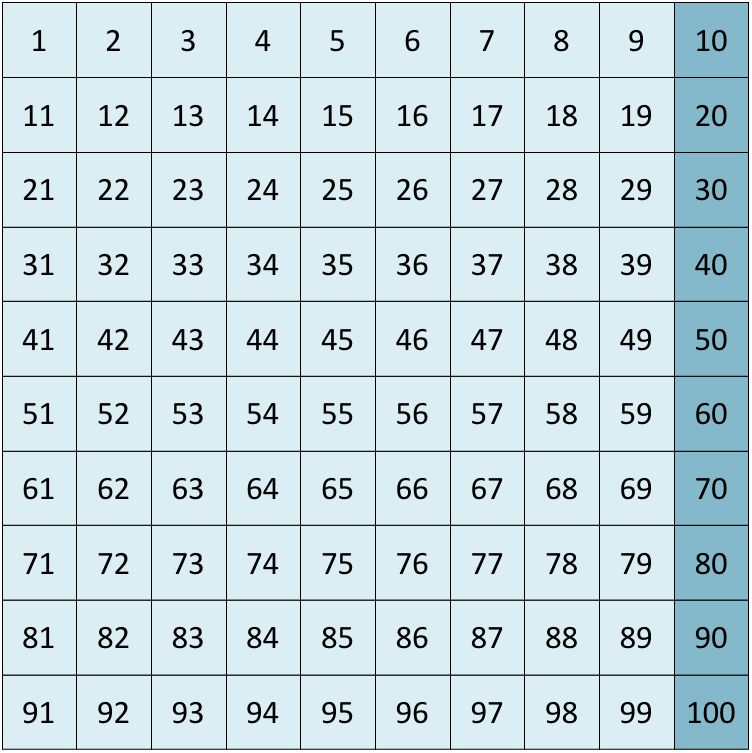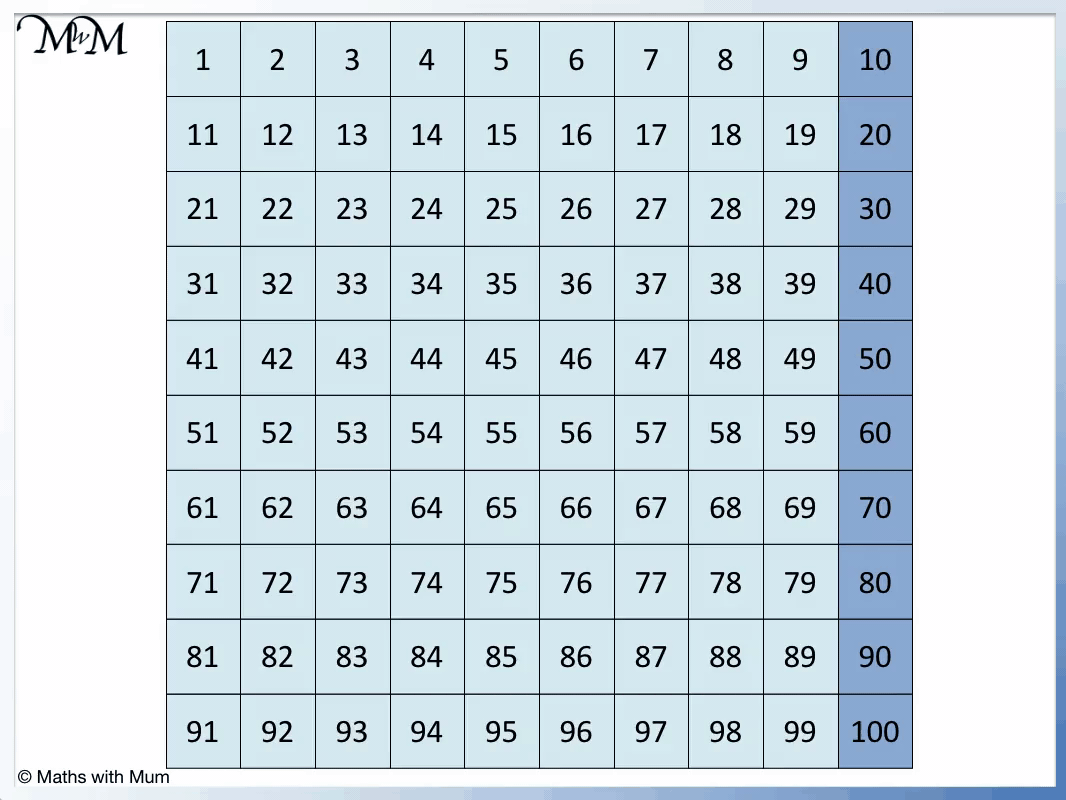# Multiples of 10

Multiples of 10• The multiples of 10 are numbers that can be divided exactly by 10, leaving no remainder.
• The first few multiples of 10 are the numbers in the 10 times table.
• For example 5 × 10 = 50 and so, the fifth multiple of ten is 50.
• For example 100 × 10 = 1000 and so, the hundredth multiple of 10 is 1000.
• All multiples of 10 have a final digit of 0.
• If a number does not have a final digit of zero, it is not a multiple of 10.

A multiple of 10 is any number that can be divided by 10 exactly, leaving no remainder.

All multiples of 10 end in a 0.#### Multiples of 10 Flashcards

Click on the multiples of 10 flashcards below to memorise the 10 times table:

×
×

=#### Multiples of 10: Interactive Questions

Multiples of 10: Interactive Questions

# Multiples of 10

## What are Multiples of 10?

Multiples of 10 are numbers that can be divided exactly by 10. They are made by multiplying any whole number by 10. A number is a multiple of 10 if its last digit is zero.

Here is a poster showing the first twelve multiples of 10:Any number in the 10 times table is a multiple of 10.

• 1 × 10 = 10
• 2 × 10 = 20
• 3 × 10 = 30
• 4 × 10 = 40
• 5 × 10 = 50
• 6 × 10 = 60
• 7 × 10 = 70
• 8 × 10 = 80
• 9 × 10 = 90
• 10 × 10 = 100
• 11 × 10 = 110
• 12 × 10 = 120

So find a multiple of ten, simply multiply a whole number by 10. For example, to find the second multiple of 10, multiply 2 by 10. 2 × 10 = 20 and so, 20 is the second multiple of 10.

## How to Find Multiples of 10

To find multiples of 10, multiply any whole number by 10. For example, 3 × 10 = 30 and so, the 3rd multiple of 10 is 30. Alternatively, multiples of 10 can be found by starting at zero and counting on in tens.

It is easy to multiply a whole number by 10. We simply place a 0 digit on the end of the number.

For example, the 345th multiple of 10 is simply 3450.

We can find multiples of 10 by counting up in tens from zero.

0 + 10 = 10, 10 + 10 = 20, 20 + 10 = 30 and so on.

When teaching multiples of 10, it can be useful to use a number grid. It is easy to count on in tens by moving one row down. All multiples of 10 on a number grid are found in the final column.We can see that each multiple of 10 is 10 away from other multiples of 10.

If you know one multiple of 10, you can find the next multiple of 10 by adding 10.

## Multiples of 10 up to 100

There are 10 multiples of 10 up to 100. They are:

10, 20, 30, 40, 50, 60, 70, 80, 90, 100.## Why do All Multiples of 10 End in 0?

All multiples of 10 end in a 0 because our numbers are written in base ten. There are only digits from 0-9 and 10 is the first number that needs two digits. Every time a number is multiplied by 10, the existing digits are carried over into a new column and a 0 is placed at the end because we have no other digits to fill the spot.Now try our lesson on Multiplying by 100 where we learn how to multiply any number by 100.error: Content is protected !!